## The image of a parabolic lens is projected onto a graph. The image crosses the x-axis at –2 and 3. The point (–1, 2) is also on the parabola

Question

The image of a parabolic lens is projected onto a graph. The image crosses the x-axis at –2 and 3. The point (–1, 2) is also on the parabola. Which equation can be used to model the image of the lens?

y = (x – 2)(x + 3)
y = (x – 2)(x + 3)
y = (x + 2)(x – 3)
y = (x + 2)(x – 3)

in progress 0
5 months 2021-09-05T09:05:08+00:00 1 Answers 3 views 0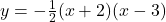Step-by-step explanation:

Given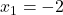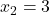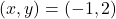— a point on the parabola

Required

The equation

First, calculate the equation from the zeros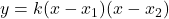Substituteand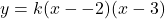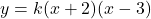To solve for k, we substitute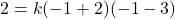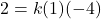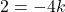Divide by -4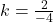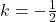So, the equation is: